Study Materials

# NCERT Solutions for Class 9th Mathematics

Page 1 of 2

## Chapter 5. Introduction To Euclid’s Geometry

### Exercise 5.1

Chapter 5. ntroduction to euclid 's geometry

Excercise : 5.1

1. Which of the following statements are true and which are false? Give reasons for your answers.

(i) Only one line can pass through a single point.

(ii) There are an infinite number of lines which pass through two distinct points.

(iii) A terminated line can be produced indefinitely on both the sides.

(iv) If two circles are equal, then their radii are equal.

(v) In Fig. 5.9, if AB = PQ and PQ = XY, then AB = XY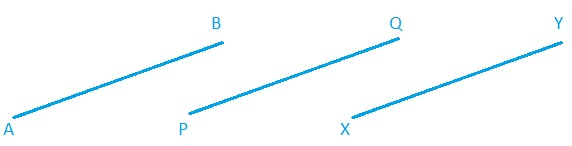Solution :

(i) false, infinite lines can pass through a point.

(ii) false, only single line can pass through two distinct point.

(iii) true, they can be produced both sides indefinitely.

(iv) true, all the circles are drawn from radius.

(v) true, all three lines are equal to each other.

2. Give a definition for each of the following terms. Are there other terms that need to be defined first? What are they, and how might you define them?

(i) parallel lines

(ii) perpendicular lines

(iii) line segment

(v) square

Solution :

(i) the two lines never meet each other and distance between them are equal.

(ii) the two lines stand on each other such that the angle between them is a right angle .

(iii) the lines which has two point.

(iv) the distance between centre and circumference.

(v) the closed figure which has all sides equal.

3. Consider two ‘postulates’ given below:

(i) Given any two distinct points A and B, there exists a third point C which is in between A and B.

(ii) There exist at least three points that are not on the same line.

Do these postulates contain any undefined terms? Are these postulates consistent? Do they follow from Euclid’s postulates? Explain.

Solution :

Yes, this postulate  contain two undefined terms that lines and point.

Yes, this postulates are inconsistent because they deal with two different situation and none of them follow the euclid’s postulate because it is based on axiom .

4. If a point C lies between two points A and B such that AC = BC, then prove that AC = AB. Explain by drawing the figure.

Solution :

Given : AC = BC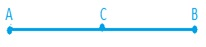To prove : AC = AB

Proof : AC +BC = AB

or         AC + AC = AB

or         2AC = AB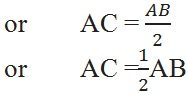5. In Question 4, point C is called a mid-point of line segment AB. Prove that every line segment has one and only one mid-point.

Solution :

C is the mid-point of AB

So,  AC = BC

Let, C` is a point on AB. Which is the mid-point of AB

So, AC` = BC`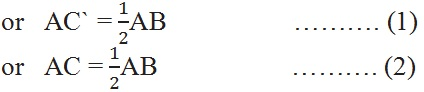By eq (1) and (2)

AC`= AC

or  C`= C

So, C and C` is same mid-point mean it is coincident.

6. In Fig. 5.10, if AC = BD, then prove that AB = CD.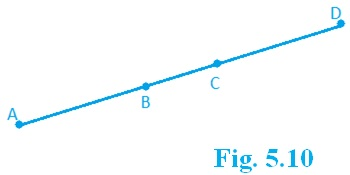Solution:

Given: AC = BD

To prove: AB = CD

Proof:  AC = BD                   ……… (1)

Subtracting BC from eq (1)

AC – BC = BD – BC

AB = CD

7. Why is Axiom 5, in the list of Euclid’s axioms, considered a ‘universal truth’? (Note that the question is not about the fifth postulate.)

Solution :

This is true in anything in any part of the world therefore, it is universal.

Page 1 of 2

Chapter Contents: